Download Presentation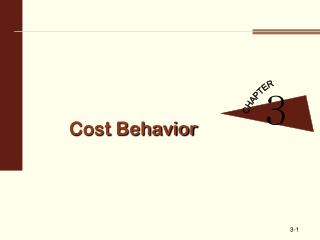Cost Behavior

# Cost Behavior - PowerPoint PPT Presentation

Cost Behavior. 3. CHAPTER. 3- 1. Basics of Cost Behavior. 1. OBJECTIVE. Cost Objects An item for which managers want cost information For manufacturing or merchandising firms, it is usually the tangible product For service firms, it is usually the service provided. 3- 2. 1. OBJECTIVE.I am the owner, or an agent authorized to act on behalf of the owner, of the copyrighted work described.
Download Presentation## Cost Behavior

An Image/Link below is provided (as is) to download presentation

Download Policy: Content on the Website is provided to you AS IS for your information and personal use and may not be sold / licensed / shared on other websites without getting consent from its author.While downloading, if for some reason you are not able to download a presentation, the publisher may have deleted the file from their server.

- - - - - - - - - - - - - - - - - - - - - - - - - - E N D - - - - - - - - - - - - - - - - - - - - - - - - - -
Presentation Transcript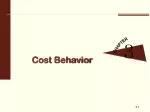### Cost Behavior

3

CHAPTER

3-1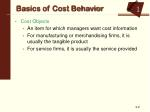Basics of Cost Behavior

1

OBJECTIVE

• Cost Objects
• An item for which managers want cost information
• For manufacturing or merchandising firms, it is usually the tangible product
• For service firms, it is usually the service provided

3-2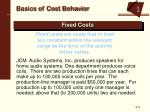1

OBJECTIVE

Basics of Cost Behavior

Fixed Costs

Fixed costs are costs that in total are constant within the relevant range as the level of the activity driver varies.

JCM Audio Systems, Inc. produces speakers for home audio systems. One department produces voice coils. There are two production lines that can each make up to 100,000 voice coils per year. The production-line manager is paid \$60,000 per year. For production up to 100,000 units only one manager is needed; above that (to 200,000 units) two are needed.

3-3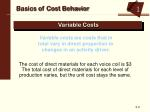1

OBJECTIVE

Basics of Cost Behavior

Variable Costs

Variable costs are costs that in total vary in direct proportion to changes in an activity driver.

The cost of direct materials for each voice coil is \$3 The total cost of direct materials for each level of production varies, but the unit cost stays the same.

3-4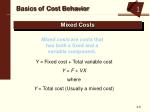1

OBJECTIVE

Basics of Cost Behavior

Mixed Costs

Mixed costs are costs that has both a fixed and a variable component.

Y = Fixed cost + Total variable cost

Y = F + VX

where

Y = Total cost (Usually a mixed cost)

3-5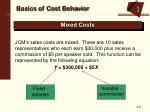1

OBJECTIVE

Basics of Cost Behavior

Mixed Costs

JCM’s sales costs are mixed. There are 10 sales representatives who each earn \$30,000 plus receive a commission of \$5 per speaker sold. This function can be represented by the following equation:

Y = \$300,000 + \$5X

Fixed - salaries

Variable - commission

3-6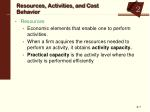2

OBJECTIVE

Resources, Activities, and Cost Behavior
• Resources
• Economic elements that enable one to perform activities.
• When a firm acquires the resources needed to perform an activity, it obtains activity capacity.
• Practical capacityis the activity level where the activity is performed efficiently

3-7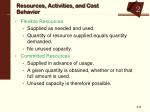2

OBJECTIVE

Resources, Activities, and Cost Behavior
• Flexible Resources
• Supplied as needed and used.
• Quantity of resource supplied equals quantity demanded.
• No unused capacity.
• Committed Resources
• Supplied in advance of usage.
• A given quantity is obtained, whether or not that full amount is used.
• Unused capacity is therefore possible.

3-8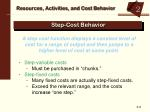2

OBJECTIVE

Resources, Activities, and Cost Behavior

Step-Cost Behavior

• A step cost function displays a constant level of cost for a range of output and then jumps to a higher level of cost at some point.
• Step-variable costs
• Must be purchased in “chunks.”
• Step-fixed costs
• Many fixed costs are actually step-fixed costs.
• Exceed the relevant range, and the costs increase “one step.”

3-9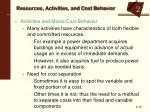2

OBJECTIVE

Resources, Activities, and Cost Behavior
• Activities and Mixed Cost Behavior
• Many activities have characteristics of both flexible and committed resources.
• For example a power department acquires buildings and equipment in advance of actual usage an in excess of immediate demands.
• However, it also acquires fuel to produce power on an as-needed basis.
• Need for cost separation
• Sometimes it is easy to spot the variable and fixed portion of a cost.
• Other times it is not: thus the need for a method to separate costs into their fixed and variable components

3-103

OBJECTIVE

Variable Component

Fixed Component

Methods of Determining Cost Behavior
• The industrial engineering method
• The account analysis method

3-114

OBJECTIVE

Quantitative Methods for Separating Mixed Costs

Y = F + VX

where:

Y = Total cost (the dependent variable)

F = Fixed cost (the intercept parameter)

V = Variable cost per unit (the slope parameter)

X = Measure of output (the independent variable)

3-122

OBJECTIVE

Quantitative Methods for Separating Mixed Costs

The High-Low Method

• Take two points (the high and the low by volume of activity) and determine the slope and intercept.
• It is objective.
• It is simple.
• The high and low points may be “outliers.”
• The high and low points may not be representative of the points in general.

3-132

OBJECTIVE

Quantitative Methods for Separating Mixed Costs

Scattergraphs

• Use a scattergraph to visually assess the relationship between cost and output.
• Allows for visual inspection of the data.
• It is subjective.
• Next let’s look at some scattergraphs.

3-144

OBJECTIVE

The Method of Least Squares – Using Excel to do Regression

• Once the data is entered:
• Choose the “Tools” menu
• Choose the “Data Analysis” option
• If this is not available you may have to manage add-ins.
• Scroll down to “Regression”
• Click on “Input Y Range” and highlight the cost cells
• Click on “Input X Range” and highlight the driver cells
• Choose your preferred location for output.
• Click ok.

3-154

OBJECTIVE

The Method of Least Squares – Using Excel to do Regression

• Interpreting the results:
• Under “coefficients” in the bottom left of the output find the intercept and the slope
• Write the equation:
• Y = 12.39X + 854.50
• Use the equation to make a point estimate:
• At a point of 350 moves the total cost is predicted to be:
• Y = 12.39(350) + 854.50
• Y = \$4336.50

3-165

OBJECTIVE

Reliability of Cost Formulas

• Hypothesis test of cost parameters
• The t Stat tests the hypothesis that the parameters are different that zero.
• The P-value is the level of significance achieved.
• Generally we are comfortable with a value of 0.05 or less.

3-175

OBJECTIVE

Reliability of Cost Formulas

• Goodness of fit
• The coefficient of determination, or R2, shows the percentage of variability in the dependent variable explained by the independent variable(s).
• The adjusted R2 takes into account how many independent variables we have used; therefore we usually prefer it to the unadjusted R2.
• The coefficient of correlation, or R, is the square root of the coefficient of determination.
• It ranges from positive to negative one.
• The higher the magnitude, the greater the correlation.

3-185

OBJECTIVE

Reliability of Cost Formulas

• Confidence Intervals

Yf ± tSe

• This is the formula for calculating a confidence interval.
• Yfis the predicted cost for a given level of activity.
• t is the t distribution (get this from the table in your book)
• Se is the standard error shown in your regression output.

3-196

OBJECTIVE

Multiple Regression
• More than one independent variable can be used to explain the dependent variable.
• Our single independent variable, number of moves, explained 85% of the variance in our dependent variable, materials handling.
• Adding another independent variable might increase the explanatory power of our model.
• Performing the regression is very similar.
• Input the data – make sure the two independent variables are side by side.
• Follow the same directions, but select both independent variable columns for the “input x range.”

3-206

OBJECTIVE

Multiple Regression
• Interpreting the results:
• Under “coefficients” in the bottom left of the output find the intercept and the slope
• Write the equation:

Y = 7.84X1 + 0.11X2 +507.31

• Examine reliability of the new model:
• Adjusted R2 is 99% - a significant improvement.
• The p-values are all very good as well.
• Note that the t statistic drops to 7 degrees of freedom because another independent variable is used.

3-217

OBJECTIVE

The Learning Curve and Nonlinear Cost Behavior
• The learning curve shows how labor hours per unit decreases as units produced increases.
• The experience curve relates cost to increased efficiency – the more you perform a task the lower the cost is of doing it.
• The cumulative average-time learning curve model states that the cumulative average time per unit decreases by a constant percentage.
• The learning rate is expressed as a percent.
• The incremental unit-time learning curve model decreases by a constant percentage each time the cumulative quantity of units produced doubles.

3-228

OBJECTIVE

Managerial Judgment
• Managerial judgment is the most widely used method in practice.
• Managers may just use their experiences and observations to determine fixed and variable costs.
• Managers may identify mixed costs and use experience to determine what part is fixed – thus denoting the rest as variable.
• This is a simple method and when the manager has a good understanding of the processes can yield good results.
• However poor judgment yields poor results.

3-23